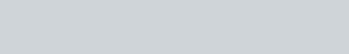In the given figure P(5, −3) and Q(3, y) are the points of trisection of the line segment
Question:

In the given figure P(5, −3) and Q(3, y) are the points of trisection of the line segment
joining A(7, −2) and B(1, −5). Then, y equals(a) 2

(b) 4

(c) $-4$

(d) $\frac{-5}{2}$

Solution:

Here, AQ : BQ = 2 : 1. Then

$y=\frac{2 \times(-5)+1 \times(-2)}{2+1}$

$=\frac{-10-2}{3}$

$=-4$

Hence, the correct answer is option (c).